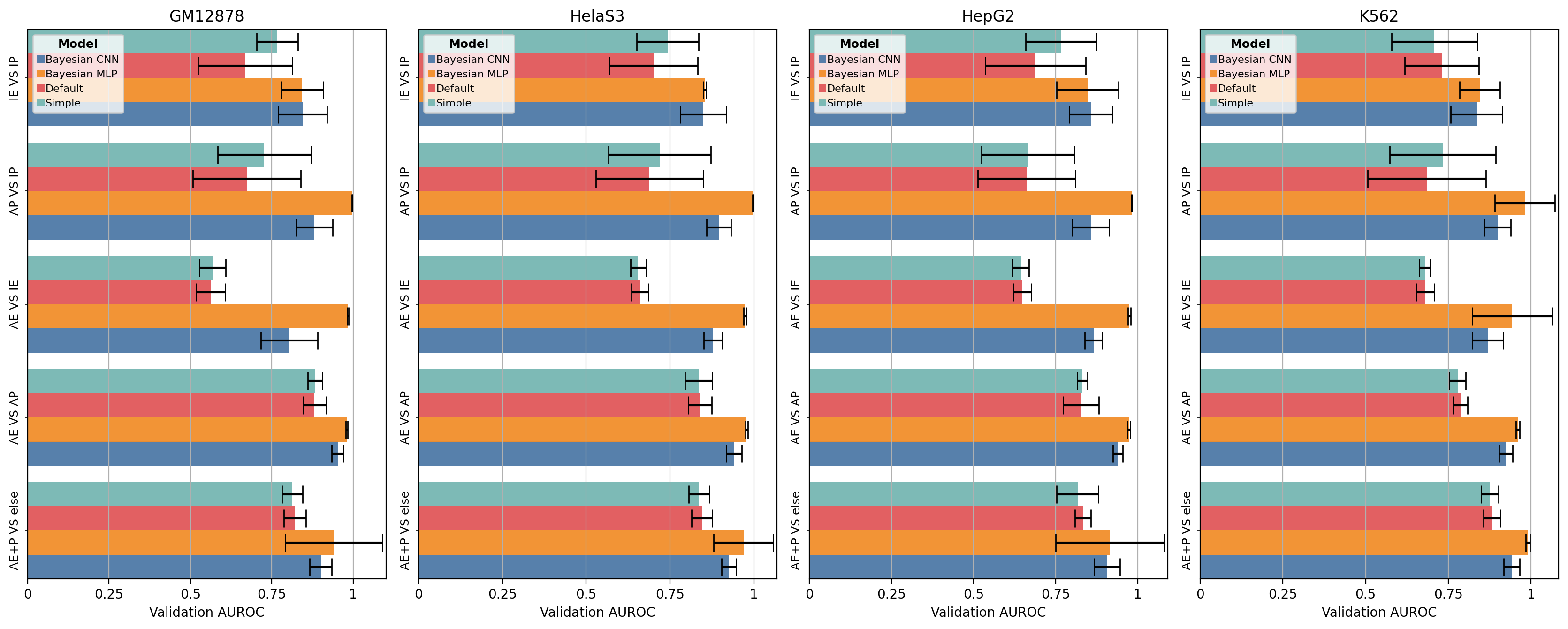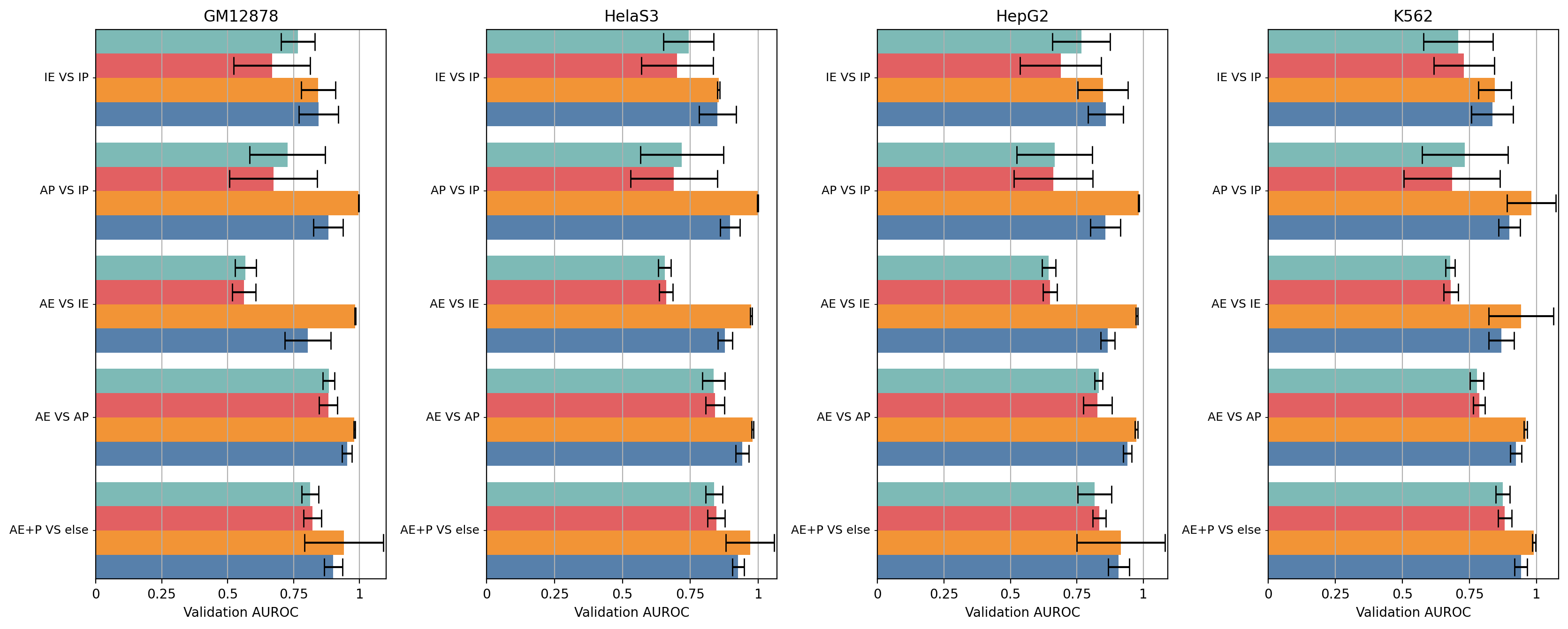Python package to easily make barplots from multi-indexed dataframes.

## Project description

Python package to easily make barplots from multi-indexed dataframes.

## How do I install this package?

pip install barplots

## Documentation

Most methods, in particular those exposed to user usage, are provided with doc strings. Consider reading these docstrings for learning the most recent updates to the library.

## Examples of the DataFrame structure

The dataframe to be provided to the barplots library may looks like the following:

miss_rate

fall_out

mcc

evaluation_type

unbalance

graph_name

normalization_name

0.0332031

0.705078

0.353357

train

10

AlligatorSinensis

0.240234

0.478516

0.289591

train

1

CanisLupus

Right Laplacian

0.0253906

0.931641

0.101643

train

100

AlligatorSinensis

Right Laplacian

0.121094

0.699219

0.220219

train

10

HomoSapiens

0.0136719

0.292969

0.722095

test

1

CanisLupus

Right Laplacian

0.0605469

0.90625

0.0622185

test

10

AmanitaMuscariaKoideBx008

0.0078125

0.4375

0.614287

train

100

AmanitaMuscariaKoideBx008

0.171875

0.869141

-0.0572194

train

100

AlligatorSinensis

0.0859375

0.810547

0.150206

train

10

MusMusculus

Right Laplacian

0.0273438

0.646484

0.415357

test

10

MusMusculus

Right Laplacian

Specifically, in this example, we may create bar plots for the features Miss rate, fallout and Matthew Correlation Coefficient by grouping on the evaluation_type, unbalance, graph_name and normalization_name columns.

An example CSV file can be seen here.

## Usage examples

Here follows a set of examples of common usages. Basically, every graph show either the same data or a mean based on the provided group by indices. Choose whetever representation is best for visualizing your data, as hardly one is better than another for every possible dataset.

N.B. The data used in the following examples are randomly generated so to be useful for test porposes. DO NOT consider these values as valid results for experiments using the same labels (cell lines etc…) which are only used to show possible usages.

For every example, the considered dataframe df is loaded as follows:

import pandas as pd

df = pd.read_csv("tests/test_case.csv")

Also, for every example, the custom_defaults used to sanitize the labels specific to the used dataset is:

custom_defaults = {
"P": "promoters",
"E": "enhancers",
"A": "active ",
"I": "inactive ",
"+": " and ",
"": "anything",
"Validation":"val"
}

### Horizontal Example A

In the following example we will plot the bars horizontally, rotating the group labels by 90 degrees and displaying the bar labels as a shared legend.

from barplots import barplots

barplots(
df,
orientation="horizontal",
show_legend=True,
minor_rotation=90,
custom_defaults=custom_defaults
)

Result can be seen here.

### Horizontal Example B

In the following example we will plot the top index as multiple subplots with horizontal bars, rotating the group labels by 90 degrees and displaying the bar labels as a shared legend.

from barplots import barplots

barplots(
df,
orientation="horizontal",
show_legend=True,
subplots=True,
minor_rotation=90,
custom_defaults=custom_defaults
)### Horizontal Example C

In the following example we will plot horizontal bars, rotating the top group labels by 90 degrees and displaying the bar labels as minor ticks.

from barplots import barplots

barplots(
df,
orientation="horizontal",
show_legend=False,
major_rotation=90,
custom_defaults=custom_defaults
)

Result can be seen here

### Horizontal Example D

In the following example we will plot the top index as multiple subplots with horizontal bars, rotating the group labels by 90 degrees and displaying the bar labels as minor ticks.

from barplots import barplots

barplots(
df,
orientation="horizontal",
show_legend=False,
major_rotation=90,
subplots=True,
custom_defaults=custom_defaults
)### Vertical Example A

In the following example we will plot the bars vertically and displaying the bar labels as a shared legend.

from barplots import barplots

barplots(
df,
orientation="vertical",
show_legend=True,
custom_defaults=custom_defaults
)

Result can be seen here

### Vertical Example B

In the following example we will plot the top index as multiple subplots with vertical bars and displaying the bar labels as a shared legend.

from barplots import barplots

barplots(
df,
orientation="vertical",
show_legend=True,
subplots=True,
custom_defaults=custom_defaults
)### Vertical Example C

In the following example we will plot vertical bars, rotating the minor group labels by 90 degrees and displaying the bar labels as minor ticks.

from barplots import barplots

barplots(
df,
orientation="vertical",
show_legend=False,
minor_rotation=90,
custom_defaults=custom_defaults
)

Result can be seen here

### Vertical Example D

In the following example we will plot the top index as multiple subplots with vertical bars, rotating the minor group labels by 90 degrees and displaying the bar labels as minor ticks.

from barplots import barplots

barplots(
df,
orientation="vertical",
show_legend=False,
minor_rotation=90,
subplots=True,
custom_defaults=custom_defaults
)## Future features

Currently it is not possible to automatically detect labels overlap and rotate them. I will work on this feature when I get the time, currently you will need to use the parameters minor_rotation and major_rotation.

## Project details

This version1.1.301.1.291.1.281.1.271.1.261.1.251.1.231.1.221.1.211.1.201.1.191.1.181.1.171.1.161.1.151.1.141.1.131.1.111.1.101.1.91.1.81.1.41.1.31.1.21.1.11.1.0

Uploaded source